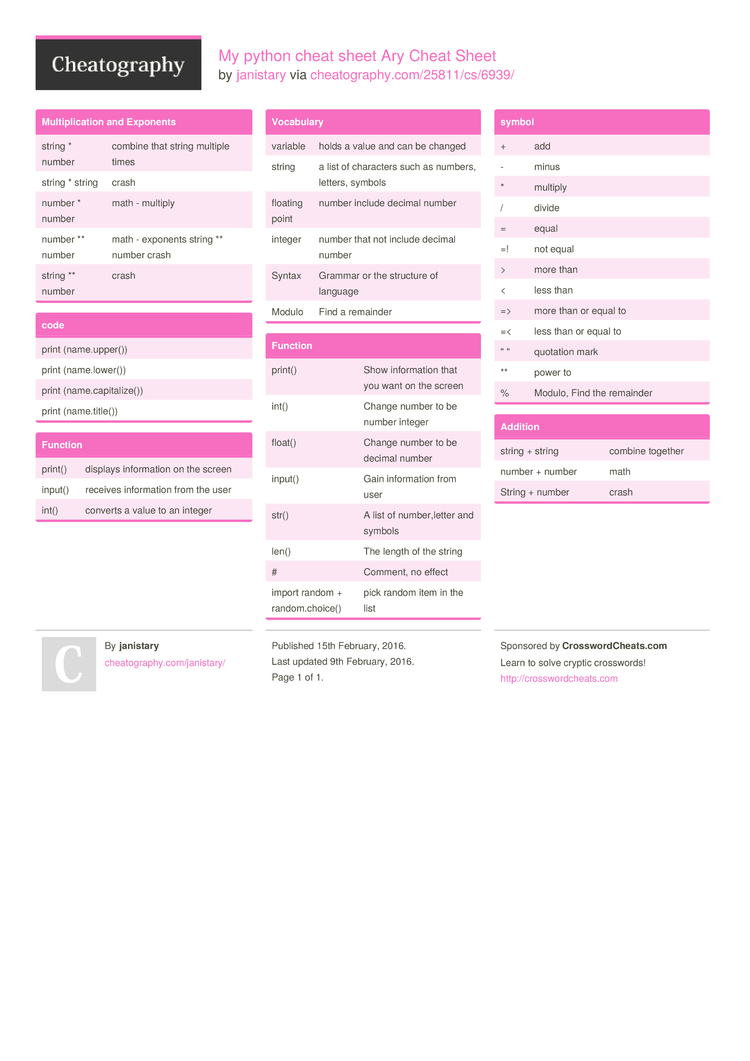# My python cheat sheet Ary Cheat Sheet by janistary

### Multip­­li­c­ation and Exponents

 string * number combine that string multiple times string * string crash number * number math - multiply number ** number math - exponents string ** number crash string ** number crash

### code

 print (name.u­pp­er()) print (name.l­ow­er()) print (name.c­ap­ita­lize()) print (name.t­it­le())

### Function

 print() displays inform­­ation on the screen input() receives inform­­ation from the user int() converts a value to an integer

### Vocabulary

 variable holds a value and can be changed string a list of characters such as numbers, letters, symbols floating point number include decimal number integer number that not include decimal number Syntax Grammar or the structure of language Modulo Find a remainder

### Function

 print() Show inform­ation that you want on the screen int() Change number to be number integer float() Change number to be decimal number input() Gain inform­ation from user str() A list of number­,letter and symbols len() The length of the string # Comment, no effect import random + random.ch­oice() pick random item in the list

### symbol

 + add - minus * multiply / divide = equal =! not equal > more than < less than => more than or equal to =< less than or equal to " " quotation mark ** power to % Modulo, Find the remainder

 string + string combine together number + number math String + number crash1 Page
//media.cheatography.com/storage/thumb/janistary_my-python-cheat-sheet-ary.750.jpg

PDF (recommended)